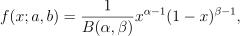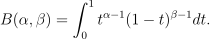/NumPy 1.14

# numpy.random.RandomState.beta

RandomState.beta(a, b, size=None)

Draw samples from a Beta distribution.

The Beta distribution is a special case of the Dirichlet distribution, and is related to the Gamma distribution. It has the probability distribution functionwhere the normalisation, B, is the beta function,It is often seen in Bayesian inference and order statistics.

Parameters: a : float or array_like of floats Alpha, non-negative. b : float or array_like of floats Beta, non-negative. size : int or tuple of ints, optional Output shape. If the given shape is, e.g., (m, n, k), then m * n * k samples are drawn. If size is None (default), a single value is returned if a and b are both scalars. Otherwise, np.broadcast(a, b).size samples are drawn. out : ndarray or scalar Drawn samples from the parameterized beta distribution.